Courses

# Three Phase Transformer Phase Groups (Part - 2) Electrical Engineering (EE) Notes | EduRev

## Electrical Engineering (EE) : Three Phase Transformer Phase Groups (Part - 2) Electrical Engineering (EE) Notes | EduRev

The document Three Phase Transformer Phase Groups (Part - 2) Electrical Engineering (EE) Notes | EduRev is a part of the Electrical Engineering (EE) Course Electrical Machines.
All you need of Electrical Engineering (EE) at this link: Electrical Engineering (EE)

Three Phase Transformer Phase Groups Part - II
In the last lecture, we have seen how to make transformer connections when the phase group such that the high voltage and the low voltage side line to phase voltages are either in phase or out of phase; that is, either they have clock number 0 or 6. Now, let us look into some transformer connection, where the clock numbers are either 1 or 11; that is the line to neutral phase voltage of the LV side leads or lags the line to neutral voltage of the HV side by plus minus 30 degree. What are the transformer connections in these two groups?

(Refer Slide Time: 01:45)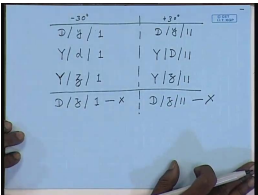For example, they can be D, y, 1 or Y, d 1 or Y, z, 1. This can also be interchanged. However, D, z, 1 is not possible. We have seen that, D, z, 1 is not possible; it can be either D, z, 0 or D, z, 6; D, z, 1 is not possible or 11 similarly, is not possible. This is minus 30 degree connection. For plus 30 degree connection, you can have D, y, 11 or Y, D, 11 or Y, z, 11. Similarly, D, z, 11 is not possible. So, let us look at… This is plus 30 degree connection. So, let us look at how we make these connections.

(Refer Slide Time: 03:29)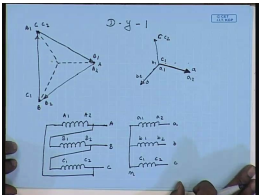Just start with D – y – 1; D – y – 1. So, the line to neutral of the high voltage side – let us say this is the line to neutral of the high voltage side. This is A; this is B; this is C. Again let us say this is the terminal A 2; this is B 2; this is C 2. Similarly, on the load voltage side, the low voltage side line to neutral voltage should… Since this is 1, it should lag the high voltage side line to neutral voltage by 30 degree. So, this should be a; this is should be b; and this should be c. Similarly, let us say these are a 2, b 2 and c 2. And obviously, these are a 1, b 1 and c 1. Hence, it is imperative that, the high voltage side line voltage a 1 a 2 be parallel to the low voltage side line to neutral voltage a 1 a 2.

So, in this case, this terminal will be A 1, so that the phasor A 1 to A 2; this is the voltage phasor A 1 to A 2 is parallel to the low voltage side voltage phasor A 1 to A 2. Similarly, B 1 B 2 should be parallel to this voltage; hence, this terminal should be B 1, so that this voltage phasor is parallel to this. And thenthis should be C 1, so that this voltage phasor is parallel to this. Hence, connection wise it will be… This is c 1 c 2. These are the terminals A, B, C; these are the terminals small a, small b, small c. A neutral point is formed by connecting a 1 b 1 c1; this is n. On the delta side, now, we see that, the connections are slightly different. Here B 1 is connected to A 2, C 1 is connected to B 2, and A 1 is connected to C 2. This is the voltage phasor diagram and the connection diagram of the phase group D – y – 1.

(Refer Slide Time: 08:25)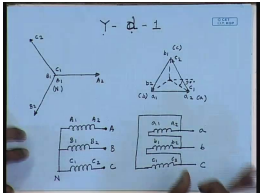Let us similarly, look at Y – d – 1; Y – d – 1 – the high voltage side. This is let us say A 1 A 2; this is B 1 B 2; this is C 1 C 2. Hence, this is the neutral point N. On the low voltage side, we have the voltages a 1 a 2, which is parallel to a 1 a 2 here. We also have the voltage b 1 b 2, which is parallel to the high voltage side voltage B 1 B 2. And the voltage c 1 c 2, which is parallel to high voltage side C 1 C 2. Hence, the line to neutral voltage of the low voltage side calling this to be terminal a; this is to be terminal b; this is to be terminal c. We see that, the line to neutral voltage on the low voltage side lags the line to neutral voltage of the high voltage side by an angle 30 degree. So, this connection gives me Y – d – 1.

Generally, for high voltage side, the representing letter is written in capital; for low voltage side, the representing letter is written in small. So, from connection wise, it will be… Neutral point is formed by connecting A 1 B 1 C 1; suppliers are given to the terminals A 2 B 2 C 2. On the low voltage side, you have again three windings: a 1 a 2, b 1 b 2, c 1 c 2. In this case, the terminal a is formed by connecting a 2 to c 1; this is terminal a.

And, terminal b is formed by connecting a 1 to b 2; this is terminal b. Terminal c is formed by connecting b 1 to c 2; this is terminal c. So, this is the connection of Y – d – 1. So, this is D – y – 1 connection; this is Y – d – 1 connection. Let us look at… These were… Both were minus 30 degree connection. Here also the line to neutral voltage phasor of the low voltage side lags the line to neutral voltage phasor of the high voltage side by an angle of 30 degree.

(Refer Slide Time: 13:07)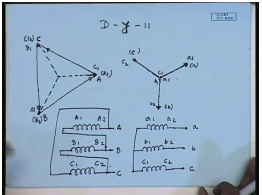Let us see some plus 30 degree connection; that is, D – y – 11 connection. Again input side, this is terminal A; this is B; this is C; it means this is A 2, B 2 and C 2. Where is the terminals A 1 B 1 C 1? That is what we will have to see. Now, here we are specifying that, this is D – y – 11. Hence, the low voltage side line to neutral voltage must lead the high voltage side line to neutral voltage by 30 degree. So, this should be the line to neutral voltage of phase b; this should be the line to neutral voltage of phase a; and this should be the line to neutral voltage of phase c. This terminal is a 2; this terminal is b 2; this terminal is c 2.

And, this terminal is a 1; this is b 1; this is c 1. Hence, the winding voltage phasor a 1 a 2 is in this direction. This should be parallel to the winding voltage phasor on the high voltage side. Hence, this terminal should be A 1, so that the line to the winding voltage phasor capital A 1 A 2 is parallel to the low voltage side line to the winding voltage phasor a 1 a 2. Similarly, this should be terminal B 1, so that capital B 1 B 2 is parallel to small b 1 b 2. This should be the terminal C 1, so that capital C 1 C 2 voltage phasor is parallel to small c 1 c 2 voltages.

The connection wise… a 1 a 2, b 1 b 2, c 1 c 2. The Y point is formed by connecting a 1 b 1 c 1; supplies are given to a 2 b 2 c 2. This is terminal a, terminal b, terminal c. On the delta side, we find that, A 2 is connected to C 1; then A 1 is connected to B 2; and B 1 is connected to C 2. This gives me… And the supplies are given to these points A, B, C. Let us compare this connection with D – y – 1. We see that, the star side connection of both of them are identical. On D – y – 1, we had connected A 2 to B 1; here we have connected A 1 to B 2; B 2 to C 1; B 1 to C 2; C 2 to A 1; C 1 to A 2. Therefore, just by interchanging these connections, a D – y – 1 transformer can be converted to a D – y – 11 transformer. Similar conclusion can be drawn for Y – d – 1. Let us look at that.

(Refer Slide Time: 19:14)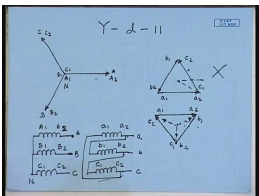Let us see the transformer connection Y – d – 11. The high voltage side is Y connected. Therefore, the line to neutral phasors are also the winding voltage phasors. Hence, this is line A or winding terminal A 2; this is line B – winding terminal B 2; this is line C – winding terminal C 2. And these are the winding terminals A 1, B 1 and C 1, which forms the star point N. On the low voltage side, the winding voltages will be in phase with the high voltage side winding voltages.

So, this will be a 1, a 2; this is will be… This will be a 1 a 2; and then this will be c 1 c 2; and this will be b 1 b 2. If we want the line to neutral voltage of the low voltage side to lead this; then this connection if we make; if this is the connection; however, this does not give Y – d – 11; it gives Y – d – 1. Hence, this connection cannot be accepted for this case. Hence, the connection will be like this – a 1 a 2, b 1 b 2 and c 1 c 2. In this case, if we make this connection; then the line to neutral voltage of the low voltage side will lead the line to neutral voltage of high voltage side and this will be a Y – d – 11 connection.

So, what will be the winding connections here? This is the high voltage winding; this is not acceptable. On the high voltage side, the star point is formed by connecting A 1 B 1 C 1. This is the neutral N. These are the line terminals A, B and C. On the low voltage side, now, we connect a 2 to b 1, b 2 to c 1 and a 1 to c 2. The lines are connected to a 2 b 2 c 2 – a, b, c.

Now, let us compare it with the connection Y – d – 1. When we compare, we see that, in this case, the high voltage side connection of both the transformers are identical. On the low voltage side, while in this case, a 1 was connected to b 2, b 1 was connected to c 2, and c 1 was connected to a 2. Here it has been reversed. Here a 2 is connected to b 1, b 2 is connected c 1, and c 2 is connected a 1. But, in both cases, the lines are obtained from the terminal a 2 b 2 c 2. So, this is the difference between Y – d – 1 and Y – d – 11 connections.

(Refer Slide Time: 26:01)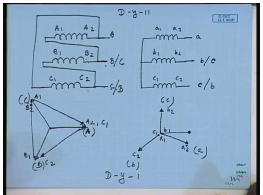Now, let us look at an interesting aspect of these connections. Let us take two connections Y – d… a connection of D – y – 11. The D – y – 11 connection was like this – A 1 was connected to B 2; B 1 was connected C 2; and C 1 was connected to A 2. And the terminals were obtained from A 2, B 2, C 2. On the low voltage side, we had… This is a 1 a 2, b 1 b 2, c 1 c 2. This was originally D – y – 11 connection. Now, let us see the supply is given to high voltage side with A phase connected here, B phase connected here, C phase connected here. Now, let us exchange two terminals: b and c of the supply; that is, instead of connecting phase B to this, if we connect phase C here; and instead of connecting phase C to this, if we connect phase B here; then let us see what happens.

So, what is the winding voltage now? This supply phase sequence is A B C; this is A; this is B; this is C. If we interchange, then what happens? The winding voltage phasor V A 1 A 2 is now the line voltage V A C. The voltage of terminal A 2 with respect to A 1 is now the line voltage V A with respect to C. So, this is the line voltage. This is A 2; this is A 1 as well as B 2; this is A 2 as well as C 1. Similarly, the winding voltage B 1 B 2; that is, B 2 with respect to B 1 is now voltage V C B – V C B. This is terminal B 1. And the winding voltage V of C 2 with respect to C 1; this is also C 2. So, this is the voltage phasor C 2 C 1. So, let us see.

Now, we have the high voltage side line voltage phasors of this. So, on the low voltage side, the voltage phasor b 1 b 2 will be… This will be b 2; this will be b 1. a 1 a 2 similarly will be… This will be a 1; this will be a 2; this will be c 1; this will be c 2. Now, let us also exchange the terminal on the low voltage side. Let us call this in the c phase and let us call this the b phase. Hence, this will be line a; this will be line b; this will be line c. On the high voltage side, this we are… this is line A; this is line B; this is line C. So, we see in this case, line to neutral voltage phasor on the high voltage side is this. This is the a phase line to neutral voltage phasor.

On the low voltage side, on the other hand, the line to neutral voltage phasor is this. Hence, the low voltage side line to neutral voltage phasor now lags the line to neutral voltage phasor of the high voltage side. Hence, this becomes effectively a D – y – 1 connection. So, we see that, we can very easily convert a D – y – 11 to D – y – 1 by simply exchanging two terminals – external terminals. There is no need to change the connection – internal connection of the transformer windings. This is sometimes useful; particularly, while connecting transformers in parallel. As we will see for parallel operations of 3 phase transformers, it is essential that, the line to neutral voltage phasor of both the transformers should be in phase.

(Refer Slide Time: 33:46)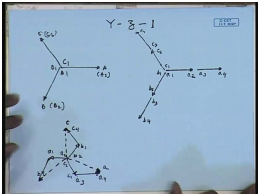Now, let us see how a zigzag connection can be handled. So, let us first take up the case of Y – z – 1. As we have seen, the D – z – 1 is not possible. So, input side is Y connected. Hence, the line to neutral voltage phasor is same as the winding voltage phasor. So, let us say this is the A phase; this is B phase; and this is C phase. This is terminal A, B, C lines. Hence, this is also A 2; this is B 2; and this is C 2. This is A 1, B 1, C 1. On the low voltage side, we will have six such phasors a 1 a 2, a 3 a 4, b 1 b 2, b 3 b 4, c 1 c 2, c 3 c 4. Since this is D z 1, the low voltage side line to neutral voltage phasor must lag the high voltage side line to neutral voltage phasor. Therefore, the line to neutral voltage phasor of the low voltage side should look… This should be c; this should be a; and this should be b.

How do we obtain this from using this set of winding voltage phasors, and with the constants that, the last leg of the winding should be made of the same phase; that is, a phase should be either a 1 a 2 or a 3 a 4. Usually, it will be a 3 a 4. So, we want to make this phase let us say using a 3 a 4; this is the phasor a 3 a 4, which is in phase with capital A 1 A 2. Hence, what should be this one? This can be… This phasor should be like this. From here we see that, it should be c 1 c 2; that is, this is c 2; this is c 1, because this is in phase opposition to this. Similarly, for b phase, we will have b 3 b 4… Hence, this phasor can be only a 1 a 2. For the c phase, this is c 4. Hence, this phasor can be b 1 b 2.

(Refer Slide Time: 38:47)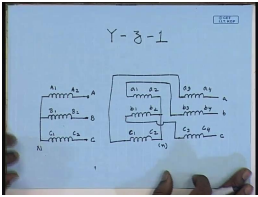As far as the connection is concerned, we have three windings on the high voltage side – A 1 A 2, B 1 B 2, C 1 C 2. The star is formed by connecting A 1 B 1 C 1. The lines are given to A 2 B 2 C 2. This is line A; this is line B; this is line C. On the zigzag side, we have six windings: a 1 a 2, a 3 a 4, b 1 b 2, b 3 b 4, c 1 c 2, c 3 c 4. The star point as we see is formed by connecting a 2 b 2 c 2. This is the star point. And lines are taken from a 4 b 4 c 4. This is line a; this is line b; this is line c. Internal connection wise, we find that, a 1 is connected to b 3, b 1 is connected to c 3, and c 1 is connected to a 3. That completes the connection of Y – z – 1. This is the Y – z – 1 transformer connection.

(Refer Slide Time: 41:36)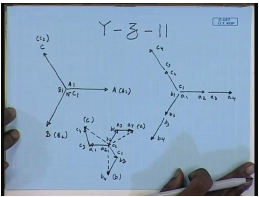So, the last connection that we will see will be the Y – z – 11 connection since Y – z – 0 or 6 is not possible. So, here again, the high voltage side is star connected; hence, the line to neutral voltage phasor is same as the winding voltage phasors. So, this is line A; this is line B; this is line C; this is the neutral point N. This is also terminal A 2; this is terminal B 2; this is terminal C 2. Hence, this is terminal A 1; this is terminal B 1; and C 1. On the low voltage side, again we have six phasors: a 1 a 2, a 3 a 4, b 1 b 2, b 3 b 4, c 1 c 2, c 3 c 4.

In order to have a Y – z – 11 connection, the low voltage side line to neutral voltage should lead the high voltage side line to neutral voltage by 30 degree. Hence, for the Y – z – 11 connection, the line to neutral voltage on the low voltage side should be like this – this should be the line b; this should be the line a; and this should be the line c. Let us see how we can obtain this kind of line to neutral voltage using this winding voltage phasor with the constant that, the last leg should be formed with the same phase voltage preferably the second set. So, this should be a 3 a 4. Hence, this phasor can only be b 2 b 1; this is b 1; this is b 2. Similarly, for b phase, this should be b 3 b 4; this should be c 2 c 1; this should be c 4 c 3; and this should be a 1 a 2.

(Refer Slide Time: 46:29)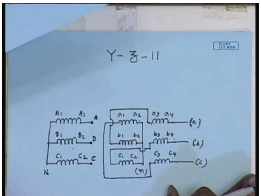Connection wise, this is the high voltage side windings. There are six windings on the low voltage side: a 1 a 2, a 3 a 4, b 1 b 2, b 3 b 4, c 1 c 2, c 3 c 4. Again, we find here that, the star point is formed by connecting a 2 b 2 c 2. From this phasor diagram, we find that, the star point is formed by connecting a 2 b 2 c 2. This is the neutral. The lines are still obtained from the terminals a 4, b 4 and c 4. This is line a; this is line b; this is line c. Now, internal connection wise, we find that, a 1 now is connected to c 3; a 1 is connected to c 3; b 1 is connected to a 3; and c 1 is connected to b 3. This completes the connection on the low voltage side. On the high voltage side, as usual, the neutral point is formed by connecting A 1 B 1 C 1; and the lines are supplied to A 2 gets A, B 2 gets B, and C 2 gets C. This is the connection diagram for Y – z – 11; where, we find that, the line to neutral voltage on the low voltage side leads the line to neutral voltage on the high voltage side by an angle of 30 degree.

It will be interesting to compare the connection of Y – z – 1 and Y – z – 11. This was the connection of Y – z – 1. We find that, the high voltage side connection on both sides, are identical. That is expected on the low voltage side in both cases; in both cases, the star point is formed by connecting a 2 b 2 c 2. However, for… As far as… Then the lines are obtained in both cases from a 4, b 4 and c 4. However, as far as the internal connections are concerned, here we see that, a 1 is connected to b 3; in this case, a 1 is connected to c 3; b 1 is connected to c 3; here b 1 is connected a 3; c 1 is connected a 3; here c 1 is connected to b 3. This is the only difference between the two connections.

The implication of this will be clear if we look at the two corresponding phasor diagrams. We see that, on the low voltage side, in both cases, the last leg of the phase is formed using a 3 a 4, b 3 b 4, c 3 c 4 in both cases. However, the initial part on the Y – z – 1 connection required c 2 c 1; whereas, Y – z – 11 connection required b 2 b 1. Hence, in Y – z – 1, the a phase is formed using a phase and c phase; while in Y – z – 11, a phase is formed using a phase and b phase; that is the difference. The rest of the connections for these two are same.

Thank you.

Offer running on EduRev: Apply code STAYHOME200 to get INR 200 off on our premium plan EduRev Infinity!

,

,

,

,

,

,

,

,

,

,

,

,

,

,

,

,

,

,

,

,

,

;